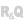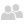• Overview
• Handling the Diagram Editor control programmatically
• Overview
• Export a diagram as an image
• Managing shape libraries
• Programming tips
• Retrieving the characteristics of a selection in a Diagram Editor control
• Using the Note property of the different shapes
• Determining the type of a shape
• WEBDEV specific features
• Programming the Diagram Editor control in WEBDEV
• Example: Getting and modifying the selected shape
• Example: Getting the index of a shape added in a Diagram Editor control
• Associated WLanguage properties
• Properties specific to the management of Diagram Editor controls
WINDEVWEBDEVWINDEV MobileOthersHandling Diagram Editor controls programmatically
Overview
In WINDEV and WEBDEV, you can handle Diagram Editor controls programmatically. To do so, use the control variable in the code.
Diagram Editor controls can also be handled programmatically using Diagram functions.
This help page explains how to handle a Diagram Editor control programmatically.
Handling the Diagram Editor control programmatically

Overview

Diagram Editor controls allow you to programmatically create and display diagrams. To do so, WLanguage provides you with:

Export a diagram as an image

To export a diagram as an image, you can use DiagramToImage. This function exports the diagram as a variable of type Image. Then, simply call one of the following functions to get the image of the diagram in the desired format:
 dSaveImageBMP Saves an image:in a file in BMP format.in memory. dSaveImageGIF Saves an image:in a file in GIF format.in memory. dSaveImageICO Saves an image in icon format either in a file, or in memory. dSaveImageJPEG Saves an image:in a JPEG file.in memory. dSaveImagePNG Saves an image:in a PNG file.in memory. dSaveImageTIFF Saves an image in TIFF format into a file or memory.

Managing shape libraries

Multiple shape libraries are included with the Diagram Editor control.
You can create your own shape library. A shape library is a diagram loaded as a library. This diagram can be created:
• via the Diagram Editor control:
1. Create a diagram.
2. Import the images that correspond to the desired shapes.
3. Save the diagram as a ".wddiag" file.
• programmatically:
1. Create a variable of type Diagram. This variable will hold the shape library.
2. Create the different custom shapes.
3. Add the shapes to the "Library" diagram.
4. Save the "Library" diagram as a ".wddiag" file.
Once the "Library" diagram has been created:
2. Add the library to the array of libraries of the final diagram.
Example of a library created programmatically:
sFile is string = fTempDir() + [ fSep ] + "custom_library.wddiag"

// Creates a library entirely through programming
TempLibrary is Diagram

D1 is diagOval
D1.Width = 50
D1.Height = 50
D1.Background.Color = DarkRed

D2 is diagOval
D2.Width = 50
D2.Height = 70
D2.Background.Color = DarkGreen

// Use the shapes of the temporary diagram to create the library
// Saves the diagram to the disk
DiagramSave(TempLibrary, sFile)

// Load the diagram as a library
MyLibrary is diagLibrary
MyLibrary.Name = "Custom"

// Adds the library to the Diagram Editor control

ToastDisplay("The custom library has been added to the list.")
Remark: To use only your custom libraries, delete the default libraries. To do so, use ArrayDeleteAll on the array of libraries of the diagram.
Programming tips

Retrieving the characteristics of a selection in a Diagram Editor control

You can retrieve the characteristics of a selection in a Diagram Editor control using the Selection property. If the selection includes more than one shape, you can loop through the array of shapes.
Example:
// Retrieve the selected elements in a Diagram Editor control
MySelection is diagSélection <- DIAGEDT_MyDiagram.Selection
IF MySelection.Shape.Count > 0 THEN
// Get the shapes
FOR EACH stShape OF MySelection.Shape
STC_SELECTION_INFO = stShape.Name + "has been selected." +
" [ " + stShape.X + ", " + stShape.Y + " - " + stShape.Width +
"x" + stShape.Height + " ]"
END
ELSE
STC_SELECTION_INFO = "Click a shape in the diagram to get the selection"
END

Using the Note property of the different shapes

diagShape variables (diagOval, diagRectangle, etc.) have a Note property. This property can be used to save additional information: shape number, type of shape, business logic information, etc, ... If the diagram is saved as a "wddiag" file, the information specified using the Note property is also saved. This information can then be read and processed when looping through the different shapes of a diagram.
To see an example of this solution, see creating an interactive diagram.

Determining the type of a shape

To determine the type of a shape, simply assign the shape to the different available variables.
Example:
FOR EACH shape OF DIAGEDT_MyDiagram.Shape
ImageShape is diagImage <- shape
IF ImageShape <> Null THEN
// The shape is an image
END
ENDWEBDEV specific features

Programming the Diagram Editor control in WEBDEV

You can handle the Diagram Editor control in server code using the different functions and types of variables available in WLanguage.
In browser code, these functions and variables are not available. The solution is to use an invisible Button control containing the server code to be executed. The click code of this Button control can be executed from the browser code using ExecuteProcess with the trtClick constant.
Remarks:
• It is recommended to use an "AJAX" server click code.
• AJAXExecute cannot be used in browser processes.
For example, the double-click browser code of a form can execute the code of the BTN_DoubleClick control:
// Double click on a shape in the Diagram Editor control
ExecuteProcess(BTN_DoubleClick, trtClick)

Example: Getting and modifying the selected shape

You can use the Selection property to get and set the characteristics of the selected shape in a Diagram Editor control in a WEBDEV site.
To do so, simply:
• Create a Button control to get the new shape.
• Run the code of the button from the "Select a shape" browser event of the Diagram Editor control.
Here is the server click code of the Button control ("Ajax" option enabled):
// Get the selection
MySelection is diagSelection <- DIAGEDT_MyDiagram.Selection
// Checks if the selection contains only one shape
IF MySelection.Shape.Count = 1 THEN
// changes the colors
MySelection.Shape.Border.Color = LightGreen
MySelection.Shape.Background.Color = LightRed
ELSE
Info("Click on a shape in the diagram to see the selection")
END
Here is the "Select a shape" browser code of the Diagram Editor control that executes the click code of the button:
ExecuteProcess(BTN_Select, trtClick)
RETURN True

Example: Getting the index of a shape added in a Diagram Editor control

You can use the "Add a shape" browser event of a Diagram Editor control in a WEBDEV site to get the index of the shape added in the control.
To do so, simply:
• Create a Button control to:
• get the mouse position.
• get the created shape.
• Run the code of the button from the "Add a shape" browser event of the Diagram Editor control.
The code of the button is as follows:
• Initialization code of BTN_Add (server):
GLOBAL
XPos, YPos are int <browser synchronized>
• Click code of BTN_Add (browser):
(XPos, YPos) = (MouseXPos(mpControl), MouseYPos(mpControl))
• "Click" server code of BTN_Add ("Ajax" option enabled):
MyDiagram is Diagram
nIndex is int

MyDiagram <- DIAGEDT_NoName1

nIndex = DiagramInfoXY(DIAGEDT_NoName1, XPos, YPos)

IF nIndex = Null = THEN
RESULT False
END

MyShape is diagShape <- diagram.Shape[nIndex]
• "Add a shape" code of the Diagram Editor control (browser):
RETURN True
Associated WLanguage properties

Properties specific to the management of Diagram Editor controls

The following properties are used to manage the characteristics of a Diagram Editor control programmatically:New in version 28EditMode The EditMode property gets and sets the editing mode of the Diagram Editor control: selection or freehand drawing. GridlinesVisible The GridlinesVisible property is used to: Determine whether or not gridlines are visible in a control. Show or hide gridlines in a control. Library The Library property allows you to handle the different preset libraries associated with a Diagram Editor control. This property accesses the array of libraries of the Diagram Editor control. LibraryPanelVisible The LibrayPanelVisible property is used to: determine if the "Library" panel is displayed in a Diagram Editor control. show or hide the "Library" panel in a Diagram Editor control. ModifierPanelVisible The ModifierPanelVisible property is used to: determine if the "Modifier" panel is displayed in a Diagram Editor control. show or hide the "Modifier" panel in a Diagram Editor control. PageBorderVisible The PageBorderVisible property is used to:determine if the page borders are displayed in a Diagram Editor control. show or hide the page borders in a Diagram Editor control. Selection The Selection property is used to get the characteristics of the selection (or cursor): in a Word Processing control.Remark: This selection is in the section being edited in the control (body, header or footer). in a Spreadsheet control.in an HTML Editor control. in a Diagram Editor control.
For a complete list of WLanguage properties that can be used with Diagram Editor controls, see Diagram Editor control properties.
Minimum version required
• Version 27